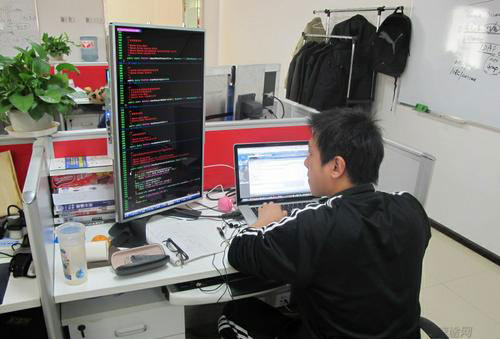# php变量的介绍 实例分析-PHP基础教程第四讲

php变量的一个简单实例：

```<?php
\$x=5;
\$y=6;
\$z=\$x+\$y;
echo \$z;
?>
```### PHP 变量规则

• 变量以 \$ 符号开头，其后是变量的名称
• 变量名称必须以字母或下划线开头
• 变量名称不能以数字开头
• 变量名称只能包含字母数字字符和下划线（A-z、0-9 以及 _）
• 变量名称对大小写敏感（\$y 与 \$Y 是两个不同的变量）

### 如何创建 PHP 变量

PHP 没有创建变量的命令，变量会在首次为其赋值时被创建。下面是实例：

```<?php
\$txt="Hello world!";
\$x=5;
\$y=10.5;
?>
```

PHP 根据它的值，自动把变量转换为正确的数据类型。

### PHP 变量作用域

PHP 有三种不同的变量作用域：

• local（局部变量）
• global（全局变量）
• static（静态变量）

Local 和 Global 作用域

```<?php
\$x=5; // 全局作用域
function myTest() {
\$y=10; // 局部作用域
echo "<p>测试函数内部的变量：</p>";
echo "变量 x 是：\$x";
echo "<br>";
echo "变量 y 是：\$x";
}
myTest();
echo "<p>测试函数之外的变量：</p>";
echo "变量 x 是：\$x";
echo "<br>";
echo "变量 y 是：\$x";
?>
```

PHP global 关键词

global 关键词用于访问函数内的全局变量。

```<?php
\$x=5;
\$y=10;
function myTest() {
global \$x,\$y;
\$y=\$x+\$y;
}
myTest();
echo \$y; // 输出 15
?>
```

PHP static 关键词

```<?php
function myTest() {
static \$x=0;
echo \$x;
\$x++;
}
myTest();
myTest();
myTest();
?>
```

0
1
2
3
4

• 彩虹堂

PHP 变量是php学习的基础